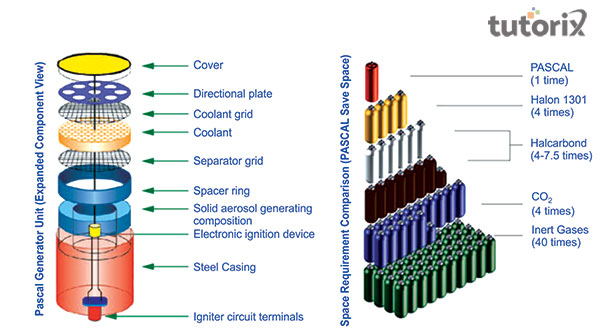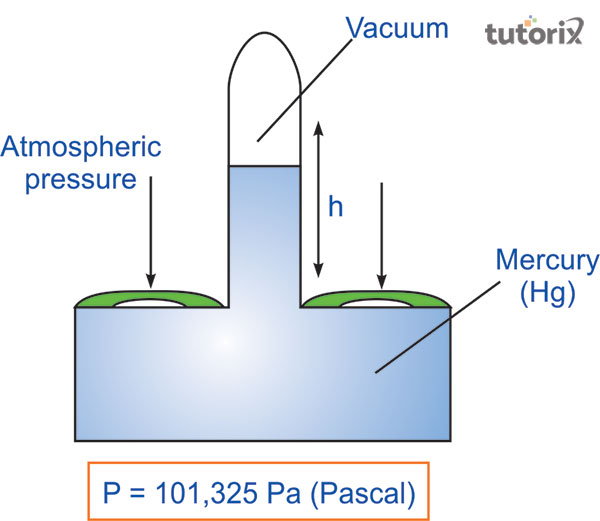# Relation Between Bar and Pascal

## Introduction

The bar is often used in order to express the atmospheric pressure. The atmospheric air that is often provided in millibars and the standard pressure is determined as the atmospheric pressure is denoted as 1013.25 mbar 101.325 kPa 1.01325 bars.

This principle is used to state the relation between Pascal and bar, where about 14.7 pound/inch2.

In this case, bar is determined as the metric unit of pressure and is not the part of SI units or the system of International Units . The bar can also be defined as the metric unit that is equivalent to 100,000 Pascal or 100 kPa. 100 kPa is less than the current average air pressure that is exerted on the earth’s surface at sea level. The air pressure that is exerted on the earth at sea level is 1.013 approx.

## What is Bar?

The bar in Greek is named Gr: Baros which means weight or mass. Between the years 1793- 1795, the term bar was utilized for unit of weight. This unit of weight is determined as the metric version of the unit system. Atm and Bar both are considered to be metric units representing atmospheric pressure. The bar is not part of the SI system but rather is the part of Metric system (Kim et al. 2018).

The formal of Bar to atm can be written as 1m2 area, that is 1 bar = 0.986923 atm.

The formula of atm to bar can be stated as 1 atm = 1.01325 bar. The US customary unit of the bar is determined as 14.50377 psi.

## What is Pascal?

Pa or Pascal is the unit of pressure that is present in the SI unit system. Pascal is named fate the famous mathematician and scientist, Blaise Pascal. Here, 1 Pascal is equal to 1 Newton (N) of the force that is put over the base of 1/m2 (Pisano, 2021).

Pascal in Physics is often determined as the pressure of 1 Newton/m2. Pascal is part of a system of SI units that are denoted as 1 kg/m/s2. The unit of Pascal is small and incontinent for several purposes.Figure 1: Pascal as Aerosol Fire Extinguishing Agent

The unit that is kPa or kilopascal of 1,000 Newton/m2 is used commonly for measuring the air pressure. The value of 1 Pascal is 1 N/m2.

The general multiple units of Pa or Pascal are the hectopascal which is 1 hPa = 100 Pa and this is equivalent to 1 millibar, 1 centibar, and also to 1 kilopascal which can be denoted as 1 kPa = 1000 Pa.

## Relation between Bar and Pascal

Pascal and bar both are considered as the units used to measure the pressure of the atmosphere. Here, the bar is defined as the metric unit that is equivalent to 100,000 Pascal or 100 kPa. 100 kPa is relatively fewer as compared to pressure of air that is exerted on the earth’s surface at sea level (Learning, 2021).

On the other side, Pascal is the unit of pressure that is present in the SI unit system and 1 Pascal is equal to 1 Newton (N) of the force that is put over the base of 1/m2.Figure 2: Barometers and Pascal’s Law

The formulas that can denote the connection between bar and pascal more prominently are

Bar to Pascal formula: 1 bar = 105 pascals and Pascal to bar formula: 1 pascal = 10-5 bar or 1 Pascal = 0.01mbar.

## Conversion of Bar and Pascal

The basic multiple units of Pa or Pascal are the hectopascal which is 1 hPa = 100 Pa and this is equivalent to 1 millibar, 1 centibar, and also to 1 kilopascal which can be denoted as 1 kPa = 1000 Pa.

The value of air pressure that can denote the conversion of Bar to Pascal is, 1bar = 100 kilopascals. As per Meteorological forecasts, it can be mentioned that the report of the atmospheric pressure is provided in hectopascals as recommended by the World Meteorological Organization (Kim et al. 2018).

Blaise Pascal had experimented with a barometer and had also contributed to hydrostatics and hydrodynamics. Pascal has been adapted for the SI unit system that is N/m2 in the year 1971, by General Conference on Measures and Weights (Kumar et al. 2019).

Pa is equivalent to the SI unit of energy density(J/m3)b and is generally applied to the thermodynamics of pressurized gas and also to the energy density of magnetic, gravitational, and electric fields.

## Conclusion

According to the Pascal law, it can be stated that when a certain amount of pressure is pertained to uniform liquids that are restricted, then those liquids will get transfer the same pressure in every direction keeping their entire rate equal. Derivation of Pa or Pascal is denoted as 1 Pa = 1 N/m2 and is termed after the famous mathematician and scientist, Blaise Pascal. 1 Pa = 1 N/m2, means that 1 Pa is equivalent to a certain amount of air pressure that is often exerted by the magnitude force of 1 Newton (N) and is perpendicular on an area of 1 m2. The pressure of water is often measured in a bar.

The factors that help to convert bar to N/mm2 can be determined as 1 N/mm2 = 1,000,000 pascals (Pa), N/mm2 value = bar value x 0.1., and 1 bar = 100,000 pascals (Pa).

## FAQs

Q1. What do you mean by 1 Pascal?

Ans. Pascal is the unit of stress that is present in the SI unit system and 1 Pascal is equal to 1 Newton(N) of the force that is applied over the area of 1/m2. 1 Pa = 1 N/m2 is the derivation of Pascal or Pa.

Q2. What do you mean by bar in force?

Ans.The bar is the non-SI unit of pressure that is illustrated as equivalent to 105

Pa or 106 dyn/cm2 in the system of the CGS unit. The bar is also equal to earth's atmospheric pressure at sea level and denoted as 100,000 Pa = 1 bar ≈ 750.0616827 Torr.

Q3. What are the values that denote the conversation between bar to pascal?

Ans.Pascal and bar both are considered as the units used to measure the pressure of the atmosphere. The values of pressure that can denote the conversion of Bar to Pascal are, 1bar = 100 kilopascals, 1 bar = 0.1 mPa (megapascal), 1mbar = 100Pa, and 1 bar = 0.986923.

Q4. How much is the conversion of kg/cm2 to bar?

Ans.The air pressure that provides the reading in kg/cm2 can be converted to the bar. This can be calculated through the formula: 1 bar = 100,000 pascals ; 1 kg/cm2 = 98,066.5 pascals; bar value x 100,000 Pa = kg/cm2 value x 98,066.5 Pascal; and the the bar value = kg/cm2 value x 0.980665.

Updated on: 13-Oct-2022

1K+ Views